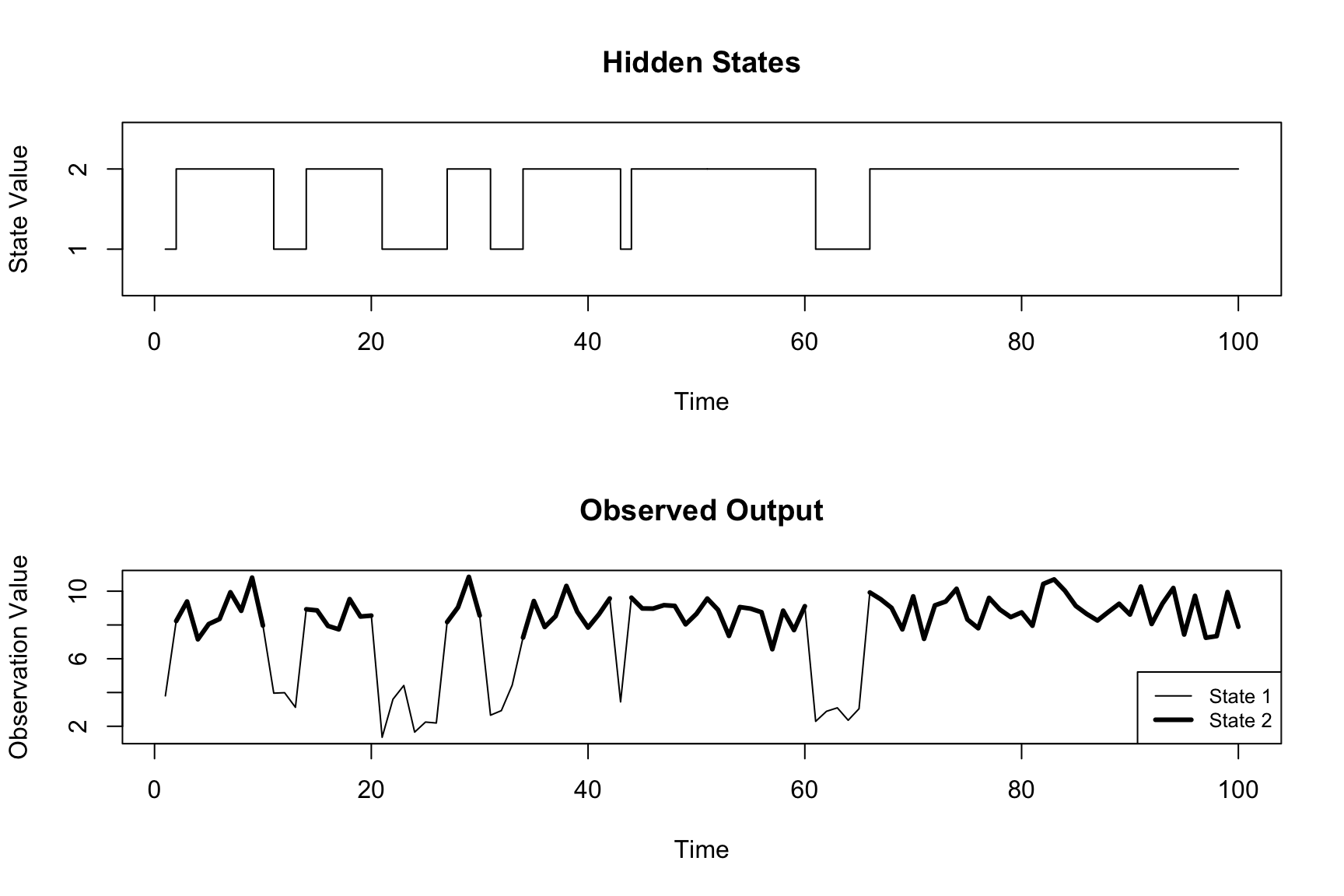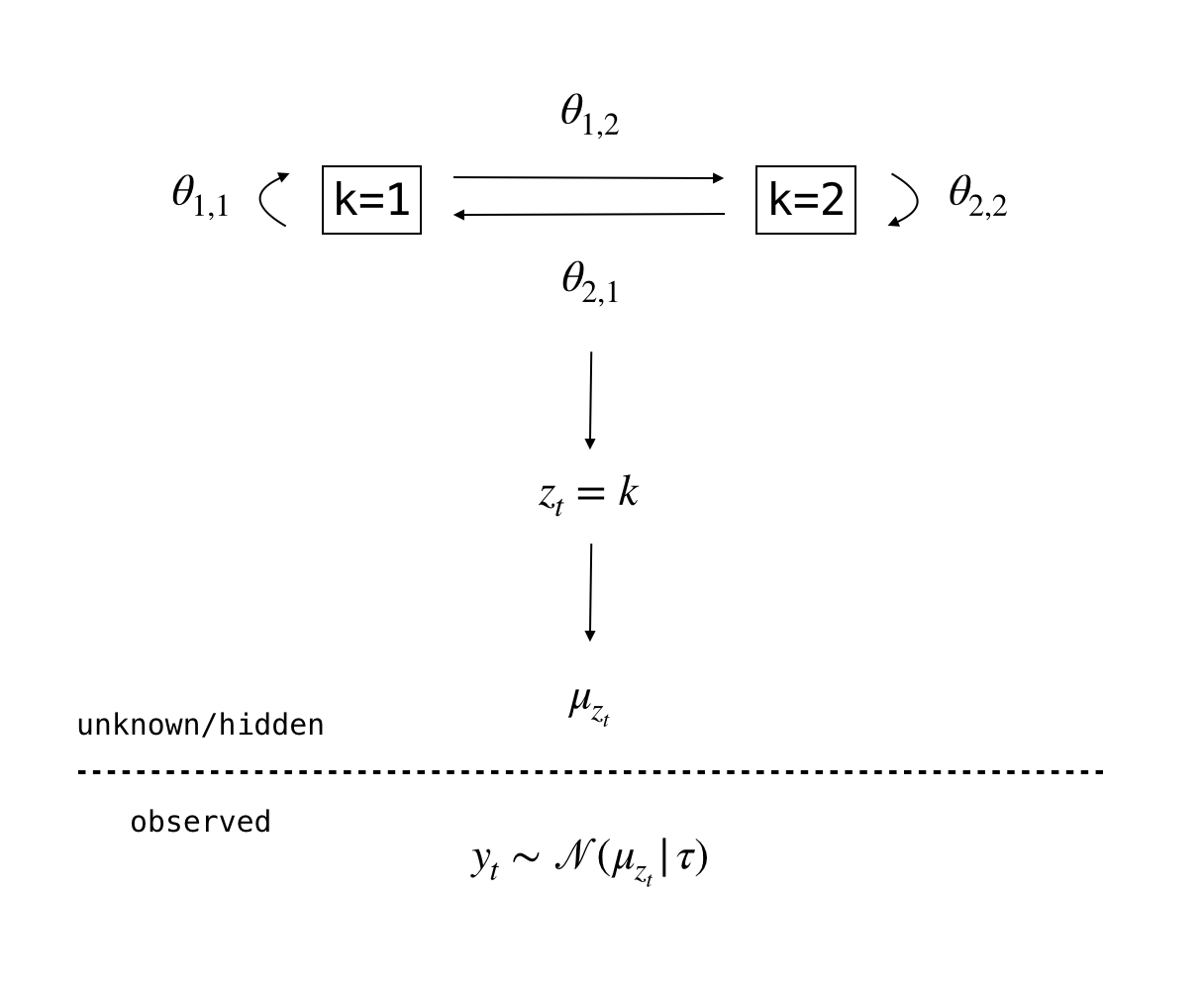## Introduction

This case study shows how we can apply Bayesian inference to Hidden Markov Models (HMMs) using Stan to extract useful information from basketball player tracking data. Specifically we show how to tag drive events and how to determine defensive assignment. Before diving into basketball data we show how to fit an HMM in Stan using a simple example. This should help build some intuition for those who are unfamiliar with HMMs and will also show how to specify an HMM using Stan.

The code used to build this document is available in the following GitHub repository: https://github.com/imadmali/bball-hmm.

## Simple HMM Example

HMMs enable you to model a series of observed values. The generative model produces an observation for each latent state, so you also have a series of states that corresponds to the series of observed values. The state series exhibits the Markov property so the value of the state at time $$t$$ only depends on the value of the state at time $$t-1$$. Often the states are hidden so the goal of inference using an HMM is to,

1. Estimate the parameters that allow you to transition from one state to the next and (given the state) the parameters involved in generating the observation.
2. Predict the most likely state sequence based on the observed data and the parameters estimated in (1).

The plot below outlines an HMM that we simulated. The model involves one sequence of observed outcomes generated from the normal distribution and two states. At each time step we are in one of the two states. Each of the states corresponds to a location parameter from the normal distribution. This means that the observed value is generated depending on which one of two location parameters is selected, which in turn depends on which state you are in. In more complicated data you could have multiple observations and many more states at each time step.

You can see how state 1 corresponds to smaller values of the outcome while state 2 corresponds to relatively higher values of the outcome. In most real-word situations you do not know the state value at each time step because it is hidden. So in order to fit the model and infer the state sequence you first need to make an assumption as to how many possible states there might be at each time step.

hmm_data <- readRDS("../data/hmm_example.RDS")
z <- hmm_data$z y <- hmm_data$y
par(mfrow=c(2,1))
plot(hmm_data$z, type="s", main = "Hidden States", ylab = "State Value", xlab = "Time", ylim = c(0.5,2.5), yaxt = "n") axis(2, 1:2, 1:2) plot(hmm_data$y, type = "l",
main = "Observed Output",
ylab = "Observation Value",
xlab = "Time")
y_plt <- hmm_data$y y_plt[hmm_data$z==1] <- NA
lines(y_plt, lwd = 3)
legend("bottomright", c("State 1","State 2"), lty = c(1,1), lwd = c(1,3), cex = 0.8)Suppose we don’t know how many states there are at each time step $$t$$, but we assume that there are two states $$z_t \in [1,2]$$. The full generative model can be defined as,

\begin{align*} &y_t \sim \mathcal{N}(\mu_{z_t}, 1) \\ &z_t \sim \mathcal{Categorical}(\theta_{z_{[t-1]}}) \\ &\mbox{priors on } \mu_k \mbox{ and } \boldsymbol{\theta}\\ \end{align*}

This says that the distribution of each observation $$y_t$$ is normal with location parameter $$\mu_{z_t}$$ and constant scale. There are two values that $$z_t$$ can take at each time step which means that there are two values of $$\mu$$. So we have $$\mu_{z_t} \in [\mu_1,\mu_2]$$. The model also says that the state variable follows the categorical distribution which is parameterized by $$\theta_{z_[t-1]}$$. Since $$z_t$$ can take two values, $$\theta_{z_[t-1]}$$ also takes two values. Given the properties of the categorical distribution $$\theta_{z_[t-1]}$$ sums to 1. This means that $$\boldsymbol{\theta}$$ is a $$2 \times 2$$ stochastic matrix (also known as a transition matrix).

A graphical representation of the model is provided below.The parameters that we want to estimate in this model are the transition probabilities ($$\boldsymbol{\theta}$$) and the parameters associated with the emission probabilities ($$\mu_1$$,$$\mu_2$$).

• Transition probabilities govern how likely it is to move from one state to another or to stay within the same state.
• Emission probabilities govern how likely the outcome was generated by that state.

We can interpret an element in the stochastic matrix $$\boldsymbol{\theta}$$ as the probability of going from the row state to the column state from time $$t-1$$ to time $$t$$. So the diagonal elements give the probability of staying in the same state, and the off-diagonal elements give the probability of transitioning from one state to the next. Continuing with our example we have,

• $$\theta_{1,1}$$: the probability of going from state 1 to state 1.
• $$\theta_{2,2}$$: the probability of going from state 2 to state 2.
• $$\theta_{1,2}$$: the probability of going from state 1 to state 2.
• $$\theta_{2,1}$$: the probability of going from state 2 to state 1.

Since the data in this example are normally distributed the emission probabilities come from the normal distribution. The emission probabilities depend on the location and scale parameter associated with each state (i.e. the emission parameters). In our model we assume the scale parameter is known and only have to estimate the location parameters $$\mu_1$$ and $$\mu_2$$ for states 1 and 2.

In order to estimate the parameters we need to define the posterior distribution which requires us to specify the likelihood of the data and the priors. The likelihood is defined by the probability of observing that particular sequence of outcome variables. Using the forward algorithm we can efficiently calculate the likelihood of the data. The forward algorithm is computing the following marginalization efficiently, $p(y | \theta, \mu) = \sum_{z} p(y,z | \theta, \mu)$

The priors are defined on the stochastic matrix and on the emission parameters. Since each row of the stochastic matrix sums to 1, a natural prior choice is the Dirichlet distribution on each row of the matrix.

We can then use Stan’s variant of the Hamiltonian Monte Carlo algorithm to estimate the stochastic matrix and emission parameters. Once we estimate the parameters we can determine the most probable state sequence that generated the sequence of observations. This can be computed with the Viterbi algorithm.

### Specifying the Model

Below we represent the HMM in Stan (also available in models/hmm_example.stan). The model {} block specifies the priors and the forward algorithm to determine the most likely state at each point in time, and the generated quantities {} block specifies the Viterbi algorithm which enables us to determine the most likely state sequence. This model was adapted from the Stan User’s Guide.

Notice that we have chosen to define $$\mu$$ as positive_ordered[K] mu instead of real mu[K]. This constraint is applied in order to enforce a strict separation between the two $$\mu$$ values associated with the two states. While in theory weak priors that order the parameters are sufficient to identify the model, in practice Stan needs better parameterization so we enforce an ordering. If we don’t do this the sampling algorithm may struggle to find convergence among the parameter chains (see Betancourt 2017 for more detail). An example of omitting the constraint specification is provided in models/hmm_example_bad.stan.

data {
int<lower=0> N;
int<lower=0> K;
real y[N];
}

parameters {
simplex[K] theta[K];
// real mu[K];
positive_ordered[K] mu;
}

model {
// priors
target+= normal_lpdf(mu | 3, 1);
target+= normal_lpdf(mu | 10, 1);
// forward algorithm
{
real acc[K];
real gamma[N, K];
for (k in 1:K)
gamma[1, k] = normal_lpdf(y | mu[k], 1);
for (t in 2:N) {
for (k in 1:K) {
for (j in 1:K)
acc[j] = gamma[t-1, j] + log(theta[j, k]) + normal_lpdf(y[t] | mu[k], 1);
gamma[t, k] = log_sum_exp(acc);
}
}
target += log_sum_exp(gamma[N]);
}
}

generated quantities {
int<lower=1,upper=K> z_star[N];
real log_p_z_star;
{
int back_ptr[N, K];
real best_logp[N, K];
for (k in 1:K)
best_logp[1, k] = normal_lpdf(y | mu[k], 1);
for (t in 2:N) {
for (k in 1:K) {
best_logp[t, k] = negative_infinity();
for (j in 1:K) {
real logp;
logp = best_logp[t-1, j] + log(theta[j, k]) + normal_lpdf(y[t] | mu[k], 1);
if (logp > best_logp[t, k]) {
back_ptr[t, k] = j;
best_logp[t, k] = logp;
}
}
}
}
log_p_z_star = max(best_logp[N]);
for (k in 1:K)
if (best_logp[N, k] == log_p_z_star)
z_star[N] = k;
for (t in 1:(N - 1))
z_star[N - t] = back_ptr[N - t + 1, z_star[N - t + 1]];
}
}

### Fitting the Model

We fit the model to the data provided above in order to estimate the parameters theta and mu along with the hidden state sequence z_star.

# code available in hmm_example.R
stan_data <- list(N = length(hmm_data$y), K = 2, y = hmm_data$y)
hmm_fit <- stan("../models/hmm_example.stan", data = stan_data, iter = 1e3, chains = 4)

### Post-estimation Validation

The post estimation steps that we take to validate are,

1. Diagnostics: Make sure that each parameter sample converged. This can be evaluated by examining the R-hat and effective sample size values for each parameter.
2. Predictions: Specifically we perform a posterior predictive check. Using the estimated parameters and the predicted state sequence we can predict multiple output sequences and see if they line up with the observed output sequence. Additionally, since we know the true state values, we can check to make sure the predicted state values line up the true state values.

We go through these steps below.

#### Diagnostics

For the transition probabilities $$\boldsymbol\theta{}$$ and the parameters associated with the emission probabilities $$\mu$$ we have the following parameter estimates.

print(hmm_fit, pars = "z_star", include = FALSE, probs = c(0.05,0.95))
## Inference for Stan model: hmm_example.
## 4 chains, each with iter=1000; warmup=500; thin=1;
## post-warmup draws per chain=500, total post-warmup draws=2000.
##
##                 mean se_mean   sd      5%     95% n_eff Rhat
## theta[1,1]      0.66    0.00 0.10    0.50    0.81  1647    1
## theta[1,2]      0.34    0.00 0.10    0.19    0.50  1647    1
## theta[2,1]      0.07    0.00 0.03    0.03    0.12  1638    1
## theta[2,2]      0.93    0.00 0.03    0.88    0.97  1638    1
## mu           3.03    0.01 0.23    2.67    3.40  1046    1
## mu           8.83    0.00 0.11    8.64    9.01  2305    1
## log_p_z_star -166.18    0.04 1.34 -168.85 -164.60   882    1
## lp__         -170.20    0.05 1.39 -173.00 -168.57   772    1
##
## Samples were drawn using NUTS(diag_e) at Sat Oct  5 14:02:48 2019.
## For each parameter, n_eff is a crude measure of effective sample size,
## and Rhat is the potential scale reduction factor on split chains (at
## convergence, Rhat=1).

With Rhat values close to 1 and a high enough effective sample size (n_eff) we can conclude that our parameter estimates converged.

We can also look at the traceplots to get an idea of what convergence among chains looks like.

mcmc_trace(as.array(hmm_fit), regex_pars = "^theta\\[|^mu\\[", facet_args = list(nrow = 2))